## Welcome to our Learn At Home Sale!

Limited-time Advanced Swift & Android book bundles, plus 50% off all books

# How To Make A Game Like Fruit Ninja With Box2D and Cocos2D – Part 2

This is a post by iOS Tutorial Team Member Allen Tan, an iOS developer and co-founder at White Widget. You can also find him on Google+ and Twitter. This is the second part of a tutorial series that shows you how to make a sprite cutting game similar to the game Fruit Ninja by Halfbrick […]

#### Version

• Other, Other, Other

This is a post by iOS Tutorial Team Member Allen Tan, an iOS developer and co-founder at White Widget. You can also find him on and Twitter.

This is the second part of a tutorial series that shows you how to make a sprite cutting game similar to the game Fruit Ninja by Halfbrick Studios.

In the first part, you learned how to create a Textured Polygon, and made a Watermelon out of it.

All the preparation you did in the first part will pay off in this second part of the series, where you’ll finally be able to cut our sprites.

As with the first part, this tutorial assumes that you are no stranger to Cocos2D and Box2D. If you are new to Cocos2D or Box2D, please check out the intro to Cocos2D and intro to Box2D tutorials on this site first.

## Getting Started

If you don’t have it already, download a copy of the sample project where you left it off in the previous tutorial.

Next, you need to make modifications to PolygonSprite’s structure so that it can handle being cut.

Open PolygonSprite.h and make the following changes:

```// Add inside the @interface
BOOL _sliceEntered;
BOOL _sliceExited;
b2Vec2 _entryPoint;
b2Vec2 _exitPoint;
double _sliceEntryTime;

```

Next, switch to PolygonSprite.mm and make the following changes:

```// Add inside the @implementation
@synthesize entryPoint = _entryPoint;
@synthesize exitPoint = _exitPoint;
@synthesize sliceEntered = _sliceEntered;
@synthesize sliceExited = _sliceExited;
@synthesize sliceEntryTime = _sliceEntryTime;

// Add inside the initWithTexture method, inside the if statement
_sliceExited = NO;
_sliceEntered = NO;
_entryPoint.SetZero();
_exitPoint.SetZero();
_sliceExited = 0;
```

Compile and check for any syntax errors.

The above code retrofits the PolygonSprite class and its subclasses with variables that store cut/slice information:

• entryPoint: The point where the slice first intersects the polygon.
• exitPoint: The point where the slice intersects the polygon for the second time.
• sliceEntered: Determines if the polygon has been intersected.
• sliceExited: Determines if there has been 1 complete slice for this polygon.
• sliceEntryTime: The exact time the slice entered the polygon. Used to eliminate swipes that are too slow to be considered as cuts.

## Intersecting Sprites Using Ray Casts

In order to cut sprites, you must be able to determine where it was cut. This is where Box2D ray casting comes in.

In ray casting, you specify a start point and an end point, and Box2D will trace along the line from start to end and tell you all the Box2D fixtures it collides with. Not only that, it can also perform a callback method telling each fixture what to do.

You’re going to use ray casts based on the player’s touch input to determine all the fixtures that the player’s touch passes by, and use the callback method to record the intersection points.

Open HelloWorldLayer.h and add the following inside the @interface:

```CGPoint _startPoint;
CGPoint _endPoint;
```

Switch to HelloWorldLayer.mm and make the following changes:

```// Add inside the draw method after kmGLPushMatrix()
ccDrawLine(_startPoint, _endPoint);

-(void)ccTouchesBegan:(NSSet *)touches withEvent:(UIEvent *)event
{
for (UITouch *touch in touches){
CGPoint location = [touch locationInView:[touch view]];
location = [[CCDirector sharedDirector] convertToGL:location];
_startPoint = location;
_endPoint = location;
}
}

- (void)ccTouchesMoved:(NSSet *)touches withEvent:(UIEvent *)event
{
for (UITouch *touch in touches){
CGPoint location = [touch locationInView:[touch view]];
location = [[CCDirector sharedDirector] convertToGL:location];
_endPoint = location;
}
}
```

The above code specifies a starting and ending point for the touch.

The start point is stored when the player touches the screen in ccTouchesBegan, and the end point moves while the player moves the touch in ccTouchesMoved.

The ccDrawLine method draws a line from the start point to the end point.

Compile and run, and draw a line on the screen: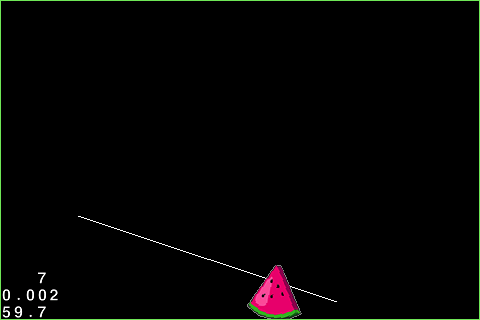This line will represent the ray cast that you will create next.

To use Box2D ray casting, you call a simple function on the world called RayCast, and provide it with the start and end points, along with a callback function that runs for each fixture the ray intersects.

The ray cast function needs to be stored in a b2RayCastCallback class.

In Xcode, go to File\New\New File, choose iOS\C and C++\Header File, and click Next. Name the new header RayCastCallback.h, and click Save.

Replace the file with the following:

```#ifndef CutCutCut_RaycastCallback_h
#define CutCutCut_RaycastCallback_h

#import "Box2D.h"
#import "PolygonSprite.h"

class RaycastCallback : public b2RayCastCallback
{
public:
RaycastCallback(){
}

float32 ReportFixture(b2Fixture *fixture,const b2Vec2 &point,const b2Vec2 &normal,float32 fraction)
{
PolygonSprite *ps = (PolygonSprite*)fixture->GetBody()->GetUserData();
if (!ps.sliceEntered)
{
ps.sliceEntered = YES;

//you need to get the point coordinates within the shape
ps.entryPoint  = ps.body->GetLocalPoint(point);

ps.sliceEntryTime = CACurrentMediaTime() + 1;
CCLOG(@"Slice Entered at world coordinates:(%f,%f), polygon coordinates:(%f,%f)", point.x*PTM_RATIO, point.y*PTM_RATIO, ps.entryPoint.x*PTM_RATIO, ps.entryPoint.y*PTM_RATIO);
}
else if (!ps.sliceExited)
{
ps.exitPoint = ps.body->GetLocalPoint(point);
ps.sliceExited = YES;

CCLOG(@"Slice Exited at world coordinates:(%f,%f), polygon coordinates:(%f,%f)", point.x*PTM_RATIO, point.y*PTM_RATIO, ps.exitPoint.x*PTM_RATIO, ps.exitPoint.y*PTM_RATIO);
}
return 1;
}
};

#endif
```

ReportFixture is the method that gets called whenever Box2D detects an intersection. You set the intersection point as the entry point if the polygon has not been intersected yet, and set it to the exit point if the polygon has already been intersected.

You convert the points using GetLocalPoint because you need to know the coordinate within the polygon’s vertices, and not the coordinate within the world. World Coordinates start from the lower left corner of the screen, while Local Coordinates start from the lower left corner of the shape.

Lastly, you return 1 to tell Box2D that this ray cast should continue to check for fixtures even after the first fixture. Returning other numbers will make the ray cast behave differently, but this is beyond the scope of this tutorial.

Switch to HelloWorldLayer.h and make the following changes:

```// Add to top of file
#import "RaycastCallback.h"

RaycastCallback *_raycastCallback;
```

Next, switch to HelloWorldLayer.mm and make these changes:

```// Add inside the init method, right after [self initSprites]
_raycastCallback = new RaycastCallback();

// Add at the end of the ccTouchesEnded method
world->RayCast(_raycastCallback,
b2Vec2(_startPoint.x / PTM_RATIO, _startPoint.y / PTM_RATIO),
b2Vec2(_endPoint.x / PTM_RATIO, _endPoint.y / PTM_RATIO));

world->RayCast(_raycastCallback,
b2Vec2(_endPoint.x / PTM_RATIO, _endPoint.y / PTM_RATIO),
b2Vec2(_startPoint.x / PTM_RATIO, _startPoint.y / PTM_RATIO));
```

You declare a RayCastCallback class and pass it as a parameter to the RayCast method. For now, you only call the RayCast when the player’s touch ends.

You do the ray cast twice because Box2D ray casting only collects fixtures in one direction and will only intersect each fixture once. To get around this, you cast one ray from the start point to the end point and one from the end point to the start point.

Compile and run. Draw a line and check the logs.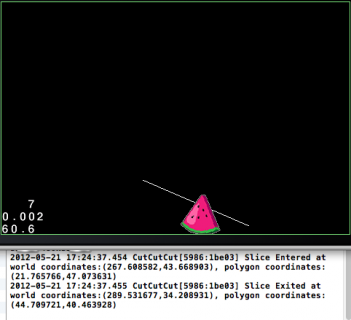## Splitting Polygons

Splitting the polygons may be the most complicated part of the tutorial, mostly because there are a lot of calculations to do, and a lot of Box2D rules to follow.

But don’t worry, this is probably the coolest part also, and I’ll walk you through it bit by bit!

Switch to HelloWorldLayer.h and make the following changes:

```// Add to top of file
#define calculate_determinant_2x2(x1,y1,x2,y2) x1*y2-y1*x2
#define calculate_determinant_2x3(x1,y1,x2,y2,x3,y3) x1*y2+x2*y3+x3*y1-y1*x2-y2*x3-y3*x1

-(b2Vec2*)arrangeVertices:(b2Vec2*)vertices count:(int)count;
-(void)splitPolygonSprite:(PolygonSprite*)sprite;
-(BOOL)areVerticesAcceptable:(b2Vec2*)vertices count:(int)count;
-(b2Body*)createBodyWithPosition:(b2Vec2)position rotation:(float)rotation vertices:(b2Vec2*)vertices vertexCount:(int32)count density:(float)density friction:(float)friction restitution:(float)restitution;
```

Switch to HelloWorldLayer.mm and add this method:

```-(void)splitPolygonSprite:(PolygonSprite*)sprite
{
//declare & initialize variables to be used for later
PolygonSprite *newSprite1, *newSprite2;

//our original shape's attributes
b2Fixture *originalFixture = sprite.body->GetFixtureList();
b2PolygonShape *originalPolygon = (b2PolygonShape*)originalFixture->GetShape();
int vertexCount = originalPolygon->GetVertexCount();

//our determinant(to be described later) and iterator
float determinant;
int i;

//you store the vertices of our two new sprites here
b2Vec2 *sprite1Vertices = (b2Vec2*)calloc(24, sizeof(b2Vec2));
b2Vec2 *sprite2Vertices = (b2Vec2*)calloc(24, sizeof(b2Vec2));
b2Vec2 *sprite1VerticesSorted, *sprite2VerticesSorted;

//you store how many vertices there are for each of the two new sprites here
int sprite1VertexCount = 0;
int sprite2VertexCount = 0;

//step 1:
//the entry and exit point of our cut are considered vertices of our two new shapes, so you add these before anything else
sprite1Vertices[sprite1VertexCount++] = sprite.entryPoint;
sprite1Vertices[sprite1VertexCount++] = sprite.exitPoint;
sprite2Vertices[sprite2VertexCount++] = sprite.entryPoint;
sprite2Vertices[sprite2VertexCount++] = sprite.exitPoint;

//step 2:
//iterate through all the vertices and add them to each sprite's shape
for (i=0; i<vertexCount; i++)
{
//get our vertex from the polygon
b2Vec2 point = originalPolygon->GetVertex(i);

//you check if our point is not the same as our entry or exit point first
b2Vec2 diffFromEntryPoint = point - sprite.entryPoint;
b2Vec2 diffFromExitPoint = point - sprite.exitPoint;

if ((diffFromEntryPoint.x == 0 && diffFromEntryPoint.y == 0) || (diffFromExitPoint.x == 0 && diffFromExitPoint.y == 0))
{
}
else
{
determinant = calculate_determinant_2x3(sprite.entryPoint.x, sprite.entryPoint.y, sprite.exitPoint.x, sprite.exitPoint.y, point.x, point.y);

if (determinant > 0)
{
//if the determinant is positive, then the three points are in clockwise order
sprite1Vertices[sprite1VertexCount++] = point;
}
else
{
//if the determinant is 0, the points are on the same line. if the determinant is negative, then they are in counter-clockwise order
sprite2Vertices[sprite2VertexCount++] = point;

}//endif
}//endif
}//endfor

//step 3:
//Box2D needs vertices to be arranged in counter-clockwise order so you reorder our points using a custom function
sprite1VerticesSorted = [self arrangeVertices:sprite1Vertices count:sprite1VertexCount];
sprite2VerticesSorted = [self arrangeVertices:sprite2Vertices count:sprite2VertexCount];

//step 4:
//Box2D has some restrictions with defining shapes, so you have to consider these. You only cut the shape if both shapes pass certain requirements from our function
BOOL sprite1VerticesAcceptable = [self areVerticesAcceptable:sprite1VerticesSorted count:sprite1VertexCount];
BOOL sprite2VerticesAcceptable = [self areVerticesAcceptable:sprite2VerticesSorted count:sprite2VertexCount];

//step 5:
//you destroy the old shape and create the new shapes and sprites
if (sprite1VerticesAcceptable && sprite2VerticesAcceptable)
{
//create the first sprite's body
b2Body *body1 = [self createBodyWithPosition:sprite.body->GetPosition() rotation:sprite.body->GetAngle() vertices:sprite1VerticesSorted vertexCount:sprite1VertexCount density:originalFixture->GetDensity() friction:originalFixture->GetFriction() restitution:originalFixture->GetRestitution()];

//create the first sprite

newSprite1 = [PolygonSprite spriteWithTexture:sprite.texture body:body1 original:NO];

//create the second sprite's body
b2Body *body2 = [self createBodyWithPosition:sprite.body->GetPosition() rotation:sprite.body->GetAngle() vertices:sprite2VerticesSorted vertexCount:sprite2VertexCount density:originalFixture->GetDensity() friction:originalFixture->GetFriction() restitution:originalFixture->GetRestitution()];

//create the second sprite
newSprite2 = [PolygonSprite spriteWithTexture:sprite.texture body:body2 original:NO];

//you don't need the old shape & sprite anymore so you either destroy it or squirrel it away
if (sprite.original)
{
[sprite deactivateCollisions];
sprite.position = ccp(-256,-256);   //cast them faraway
sprite.sliceEntered = NO;
sprite.sliceExited = NO;
sprite.entryPoint.SetZero();
sprite.exitPoint.SetZero();
}
else
{
world->DestroyBody(sprite.body);
[self removeChild:sprite cleanup:YES];
}
}
else
{
sprite.sliceEntered = NO;
sprite.sliceExited = NO;
}

//free up our allocated vectors
free(sprite1VerticesSorted);
free(sprite2VerticesSorted);
free(sprite1Vertices);
free(sprite2Vertices);
}
```

Wow, that’s a lot to take in at once. Compile and make sure your syntax is correct, then let’s tackle this method step by step:

Preparation Step
Declares the variables. The most important thing here is that you declare two new PolygonSprites, and two arrays that will store their polygon’s vertices.

Step 1
You start populating the array of vertices for each shape by adding the intersection points to both arrays.

This makes sense when you visualize cutting a polygon: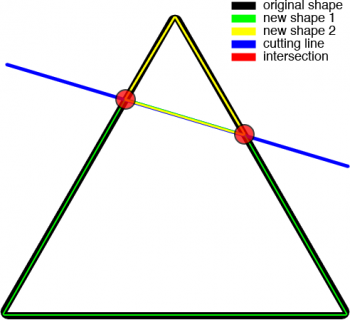The intersection points are present as vertices in both shapes.

Step 2
You assign the remaining vertices of the original shape. You know that the shape will always be cut into two parts, and the two new shapes will be on opposite sides of the cutting line.

You just need a rule to determine which shape each of the original polygon’s points should belong to.

Imagine you had a way to tell if any given three points were a clockwise rotation, or a counterclockwise rotation. If you had that, you could take the start point, end point, and one of the original points in the polygon and say:

“If these points are a clockwise rotation, add the original point to shape 2. Otherwise, add shape 1!”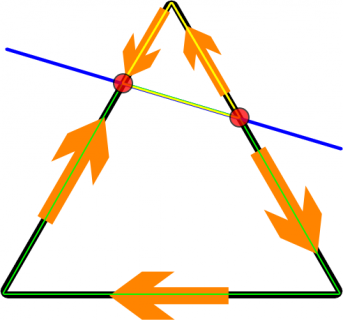Well good news – there’s a way to “determine” this, by using a mathematical concept called determinants!

In Geometry, determinants are “mathemagical” functions that can determine the direction a line takes to move from one point to another based on its resulting sign (positive, negative, or 0).

You use the determinant equation defined in HelloWorldLayer.h, and plug in the coordinates of our entry point, exit point, and each of the original vertices.

If the result is positive, then the 3 points are in clockwise order. If it is negative, then the 3 points are in counter-clockwise order. If the result happens to be 0, then the 3 points are on the same line.

You add all points clockwise to the first sprite, and the rest to the second sprite.

Step 3
Box2D expects vertices to be arranged in a counter-clockwise order, so you rearrange the vertices for the two new sprites using the arrangeVertices method.

Step 4
This makes sure that the arranged vertices adhere to all of Box2D’s rules on defining polygons. If the areVerticesAcceptable method decides that the vertices are unacceptable, then it removes the slice information from the original sprite.

Step 5
This initializes two new PolygonSprites and creates their Box2D body using the createBody method. The new sprites inherit properties from the original sprite.

If an original sprite is cut, it is reset and stored away. If a piece is cut, then it is destroyed and removed from the scene.

Whew…still with me? Good. There’s just a few more things to add before you run the program!

Still in HelloWorldLayer.mm, make the following changes:

```// Add before the @implementation
int comparator(const void *a, const void *b) {
const b2Vec2 *va = (const b2Vec2 *)a;
const b2Vec2 *vb = (const b2Vec2 *)b;

if (va->x > vb->x) {
return 1;
} else if (va->x < vb->x) {
return -1;
}
return 0;
}

-(b2Body*)createBodyWithPosition:(b2Vec2)position rotation:(float)rotation vertices:(b2Vec2*)vertices vertexCount:(int32)count density:(float)density friction:(float)friction restitution:(float)restitution
{
b2BodyDef bodyDef;
bodyDef.type = b2_dynamicBody;
bodyDef.position = position;
bodyDef.angle = rotation;
b2Body *body = world->CreateBody(&bodyDef);

b2FixtureDef fixtureDef;
fixtureDef.density = density;
fixtureDef.friction = friction;
fixtureDef.restitution = restitution;

b2PolygonShape shape;
shape.Set(vertices, count);
fixtureDef.shape = &shape;
body->CreateFixture(&fixtureDef);

return body;
}

-(b2Vec2*)arrangeVertices:(b2Vec2*)vertices count:(int)count
{
float determinant;
int iCounterClockWise = 1;
int iClockWise = count - 1;
int i;

b2Vec2 referencePointA,referencePointB;
b2Vec2 *sortedVertices = (b2Vec2*)calloc(count, sizeof(b2Vec2));

//sort all vertices in ascending order according to their x-coordinate so you can get two points of a line
qsort(vertices, count, sizeof(b2Vec2), comparator);

sortedVertices = vertices;
referencePointA = vertices;          //leftmost point
referencePointB = vertices[count-1];    //rightmost point

//you arrange the points by filling our vertices in both clockwise and counter-clockwise directions using the determinant function
for (i=1;i<count-1;i++)
{
determinant = calculate_determinant_2x3(referencePointA.x, referencePointA.y, referencePointB.x, referencePointB.y, vertices[i].x, vertices[i].y);
if (determinant<0)
{
sortedVertices[iCounterClockWise++] = vertices[i];
}
else
{
sortedVertices[iClockWise--] = vertices[i];
}//endif
}//endif

sortedVertices[iCounterClockWise] = vertices[count-1];
return sortedVertices;
}

-(BOOL)areVerticesAcceptable:(b2Vec2*)vertices count:(int)count
{
return YES;
}
```

Here is a breakdown of the methods you just created:

• createBody: This creates active Box2D bodies that can instantly collide with one another.
• arrangeVertices: This arranges vertices in a counter-clockwise order. It uses a qsort function to arrange them in ascending order according to x-coordinates, then uses determinants to make the final arrangement.
• comparator: This is the function used by qsort. It does the comparison and gives the result to qsort.
• areVerticesAcceptable: For now, it assumes all vertices are acceptable.

That's it! In theory, you can now split a polygon into two pieces. But wait..it would help to use the methods you just created! :]

Still in HelloWorldLayer.mm, add these changes:

```// Add this method
-(void)checkAndSliceObjects
{
double curTime = CACurrentMediaTime();
for (b2Body* b = world->GetBodyList(); b; b = b->GetNext())
{
if (b->GetUserData() != NULL) {
PolygonSprite *sprite = (PolygonSprite*)b->GetUserData();

if (sprite.sliceEntered && curTime > sprite.sliceEntryTime)
{
sprite.sliceEntered = NO;
}
else if (sprite.sliceEntered && sprite.sliceExited)
{
[self splitPolygonSprite:sprite];
}
}
}
}

// Add this in the update method
[self checkAndSliceObjects];
```

Compile and run, and try cutting your Watermelon.

It works! Who knew Math could cut fruits!

Note: Don't worry if the game suddenly crashes. It will be fixed once you implement the areVerticesAcceptable method.

## A Better Swipe Technique

The slicing feels a little unnatural right now, because the player can move their finger in a curve, but we're treating the cut as a straight line from where the touch started. It's also a bit weird because the cut doesn't take effect until the player lifts their finger.

To fix this, go to HelloWorldLayer.mm and make the following changes:

```// Add this method
-(void)clearSlices
{
for (b2Body* b = world->GetBodyList(); b; b = b->GetNext())
{
if (b->GetUserData() != NULL) {
PolygonSprite *sprite = (PolygonSprite*)b->GetUserData();
sprite.sliceEntered = NO;
sprite.sliceExited = NO;
}
}
}

// Add this at the end of ccTouchesMoved
if (ccpLengthSQ(ccpSub(_startPoint, _endPoint)) > 25)
{
world->RayCast(_raycastCallback,
b2Vec2(_startPoint.x / PTM_RATIO, _startPoint.y / PTM_RATIO),
b2Vec2(_endPoint.x / PTM_RATIO, _endPoint.y / PTM_RATIO));

world->RayCast(_raycastCallback,
b2Vec2(_endPoint.x / PTM_RATIO, _endPoint.y / PTM_RATIO),
b2Vec2(_startPoint.x / PTM_RATIO, _startPoint.y / PTM_RATIO));
_startPoint = _endPoint;
}

// Remove these from ccTouchesEnded
world->RayCast(_raycastCallback,
b2Vec2(_startPoint.x / PTM_RATIO, _startPoint.y / PTM_RATIO),
b2Vec2(_endPoint.x / PTM_RATIO, _endPoint.y / PTM_RATIO));

world->RayCast(_raycastCallback,
b2Vec2(_endPoint.x / PTM_RATIO, _endPoint.y / PTM_RATIO),
b2Vec2(_startPoint.x / PTM_RATIO, _startPoint.y / PTM_RATIO));

[self clearSlices];
```

You transfer the RayCast from ccTouchesEnded to ccTouchesMoved so that polygons could be split while moving the touch. Box2D ray casts can't be made too often, nor too short, so you only perform ray casts at every 5 points distance between the start and end points.

The above way of comparing distance is just an optimized way of saying "if (distance > 5)". Solving for distance requires a square root operation, and this is an expensive operation to be doing too often, so you just square both sides.

Once a ray is cast, you treat the end point of the ray cast as the new start point. Lastly, you clear all intersections whenever the player stops touching the screen.

Compile and run, and now the swipe should feel more natural.With this method, you are more prone to violating Box2D's rules. Try creating a cut that exits the sprite from the same side it enters and see what happens. Also try cutting the polygon into itty bitty little pieces and see just how small you can go.

To address these issues, switch to RaycastCallback.h, and make these changes:

```// Remove the CCLOG commands

// Add to top of file
#define collinear(x1,y1,x2,y2,x3,y3) fabsf((y1-y2) * (x1-x3) - (y1-y3) * (x1-x2))

// Remove this line from the else if statement
ps.sliceExited = YES;

// Add this inside the else if statement, right after setting the exitPoint
b2Vec2 entrySide = ps.entryPoint - ps.centroid;
b2Vec2 exitSide = ps.exitPoint - ps.centroid;

if (entrySide.x * exitSide.x < 0 || entrySide.y * exitSide.y < 0)
{
ps.sliceExited = YES;
}
else {
//if the cut didn't cross the centroid, you check if the entry and exit point lie on the same line
b2Fixture *fixture = ps.body->GetFixtureList();
b2PolygonShape *polygon = (b2PolygonShape*)fixture->GetShape();
int count = polygon->GetVertexCount();

BOOL onSameLine = NO;
for (int i = 0 ; i < count; i++)
{
b2Vec2 pointA = polygon->GetVertex(i);
b2Vec2 pointB;

if (i == count - 1)
{
pointB = polygon->GetVertex(0);
}
else {
pointB = polygon->GetVertex(i+1);
}//endif

float collinear = collinear(pointA.x,pointA.y, ps.entryPoint.x, ps.entryPoint.y, pointB.x,pointB.y);

if (collinear <= 0.00001)
{
float collinear2 = collinear(pointA.x,pointA.y,ps.exitPoint.x,ps.exitPoint.y,pointB.x,pointB.y);
if (collinear2 <= 0.00001)
{
onSameLine = YES;
}
break;
}//endif
}//endfor

if (onSameLine)
{
ps.entryPoint = ps.exitPoint;
ps.sliceEntryTime = CACurrentMediaTime() + 1;
ps.sliceExited = NO;
}
else {
ps.sliceExited = YES;
}//endif
}
```

Before accepting an exit point, the callback now checks the location of the two points. If the entry and exit points lie on opposite sides from the center of the polygon, then the cut is acceptable.

If not, you check if the entry and exit points lie on the same line by using a collinear checking function on all the lines formed by the vertices. If they are collinear, it means the intersection point is another entry point, otherwise, it is a complete slice.

Switch back to HelloWorldLayer.mm and replace the areVerticesAcceptable method with this:

```-(BOOL)areVerticesAcceptable:(b2Vec2*)vertices count:(int)count
{
//check 1: polygons need to at least have 3 vertices
if (count < 3)
{
return NO;
}

//check 2: the number of vertices cannot exceed b2_maxPolygonVertices
if (count > b2_maxPolygonVertices)
{
return NO;
}

//check 3: Box2D needs the distance from each vertex to be greater than b2_epsilon
int32 i;
for (i=0; i<count; ++i)
{
int32 i1 = i;
int32 i2 = i + 1 < count ? i + 1 : 0;
b2Vec2 edge = vertices[i2] - vertices[i1];
if (edge.LengthSquared() <= b2_epsilon * b2_epsilon)
{
return NO;
}
}

//check 4: Box2D needs the area of a polygon to be greater than b2_epsilon
float32 area = 0.0f;

b2Vec2 pRef(0.0f,0.0f);

for (i=0; i<count; ++i)
{
b2Vec2 p1 = pRef;
b2Vec2 p2 = vertices[i];
b2Vec2 p3 = i + 1 < count ? vertices[i+1] : vertices;

b2Vec2 e1 = p2 - p1;
b2Vec2 e2 = p3 - p1;

float32 D = b2Cross(e1, e2);

float32 triangleArea = 0.5f * D;
area += triangleArea;
}

if (area <= 0.0001)
{
return NO;
}

//check 5: Box2D requires that the shape be Convex.
float determinant;
float referenceDeterminant;
b2Vec2 v1 = vertices - vertices[count-1];
b2Vec2 v2 = vertices - vertices;
referenceDeterminant = calculate_determinant_2x2(v1.x, v1.y, v2.x, v2.y);

for (i=1; i<count-1; i++)
{
v1 = v2;
v2 = vertices[i+1] - vertices[i];
determinant = calculate_determinant_2x2(v1.x, v1.y, v2.x, v2.y);
//you use the determinant to check direction from one point to another. A convex shape's points should only go around in one direction. The sign of the determinant determines that direction. If the sign of the determinant changes mid-way, then you have a concave shape.
if (referenceDeterminant * determinant < 0.0f)
{
//if multiplying two determinants result to a negative value, you know that the sign of both numbers differ, hence it is concave
return NO;
}
}
v1 = v2;
v2 = vertices-vertices[count-1];
determinant = calculate_determinant_2x2(v1.x, v1.y, v2.x, v2.y);
if (referenceDeterminant * determinant < 0.0f)
{
return NO;
}
return YES;
}
```

You do 5 checks to determine if a polygon is acceptable by Box2D standards:

• Check 1: A polygon needs to have at least 3 vertices.
• Check 2: A polygon cannot exceed the predefined b2_maxPolygonVertices, which is 8 vertices.
• Check 3: The distance from each vertex must be greater than b2_epsilon.
• Check 4: The area of the polygon must be greater than b2_epsilon. This is still too small for us, so you just limit the area to 0.0001.
• Check 5: The shape must be convex.

The implementation of the first two checks are pretty straightforward, while the third and fourth checks are taken straight from the Box2D library. The last check uses determinants once again.

A convex shape's vertices should always turn in the same direction. If the direction suddenly changes, then the shape is automatically concave. You traverse the vertices of the polygon and compare the sign of the determinant. If the sign suddenly changes, then it means the vertex changed directions.

Compile and run, and make yourself some fruit salad!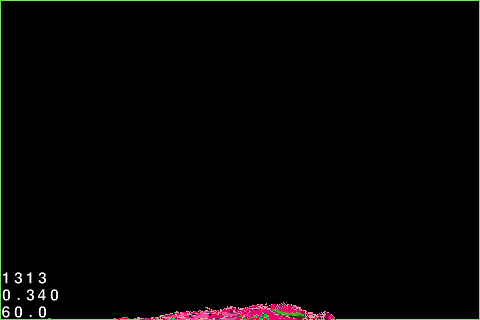## Exit Debug Mode

You're certain that the Box2D part works as expected, so you won't be needing debug drawing anymore.

Still in HelloWorldLayer.mm, make the following changes:

```// Comment these out from the draw method
ccDrawLine(_startPoint, _endPoint);
world->DrawDebugData();

// Add inside the init method
[self initBackground];

-(void)initBackground
{
CGSize screen = [[CCDirector sharedDirector] winSize];
CCSprite *background = [CCSprite spriteWithFile:@"bg.png"];
background.position = ccp(screen.width/2,screen.height/2);
}
```

Compile and run, and you should see the neat background Vicki made for this tutorial.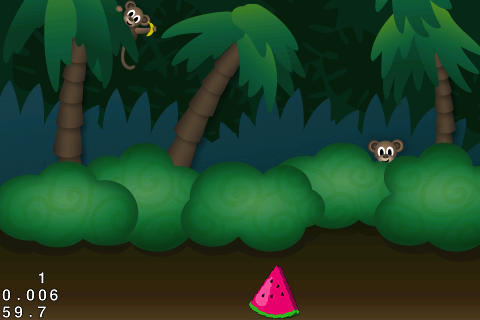## Visualizing The Swipe Using CCBlade

Without debug drawing, you need a new way to show the swipes. The CCBlade effect made by Ngo Duc Hiep is a perfect replacement.

CCBlade is maintained by a third party, so the version may differ with what this tutorial uses if it is updated. You can get the exact version of CCBlade the tutorial uses in the Classes folder of the resource kit.

You need to update CCBlade for Cocos2D 2.X, so go to CCBlade.m, rename it to CCBlade.mm, and make the following changes:

```// Replace everything starting from glDisableClientState in the draw method with this
CC_NODE_DRAW_SETUP();

ccGLBlendFunc( CC_BLEND_SRC, CC_BLEND_DST );

ccGLBindTexture2D( [_texture name] );
glVertexAttribPointer(kCCVertexAttrib_Position, 2, GL_FLOAT, GL_FALSE, sizeof(vertices), vertices);
glVertexAttribPointer(kCCVertexAttrib_TexCoords, 2, GL_FLOAT, GL_FALSE, sizeof(coordinates), coordinates);
glDrawArrays(GL_TRIANGLE_STRIP, 0, 2*[path count]-2);

// Add inside the initWithMaximumPoint method

// Remove from the setWidth method
* CC_CONTENT_SCALE_FACTOR()

// Remove from the push method
if (CC_CONTENT_SCALE_FACTOR() != 1.0f) {
v = ccpMult(v, CC_CONTENT_SCALE_FACTOR());
}
```

You made the same conversions to the drawing code as you did for PRFilledPolygon before, and removed the scale multiplier from the code since the shader program handles this now.

CCBlade populates a path array with points, and draws a textured line across those points. Right now, it updates this array in the draw method. However, it is recommended that you only do drawing calls in the draw method, and do everything else in the update method.

To better manage the path array, you will update it in HelloWorldLayer's update method.

```@property(nonatomic,retain)NSMutableArray *path;
```

```@synthesize path;
```

Next, switch to HelloWorldLayer.h and make these changes:

```// Add to top of file

float _deltaRemainder;

```

Finally, switch to HelloWorldLayer.mm and make the following changes:

```// Add inside the @implementation

// Add inside init, after _raycastCallback
_deltaRemainder = 0.0;
CCTexture2D *texture = [[CCTextureCache sharedTextureCache] addImage:@"streak.png"];

for (int i = 0; i < 3; i++)
{

}

// Add inside update, right after [self checkAndSliceObjects]
if ([_blade.path count] > 3) {
_deltaRemainder+=dt*60*1.2;
int pop = (int)roundf(_deltaRemainder);
_deltaRemainder-=pop;
}

{
{
break;
}
}

```

You make a property for the path array so you can access it from HelloWorldLayer. You then create 3 interchangeable CCBlades to be used in the game. For each blade, you set it with 50 maximum points so they don't become too long, and assign a streak texture that should already be in your Resources folder.

You set each blade's autoDim variable to NO. CCBlade uses the term "Dim" to mean that the blade effect should fade from the tail end towards the head by popping points out of the array one by one. With this, CCBlade automatically removes points from the path array.

This is quite convenient, but since CCBlade does its auto-popping feature inside its draw method, it's better to set this to NO and control the dimming ourselves in the update method.

When the player touches the screen, you assign an unused CCBlade, and the touch pushes in location points to its path array.

Last, you tell the active CCBlade to dim itself when the player stops touching the screen.

You make the update method handle the dimming of the active CCBlade. You want it to fade at the same pace no matter what the fps is, so you multiply it with delta time and a constant value.

Since our delta time will not always be a whole number, you store the remainder for use in the next cycle to make the popping rate more constant.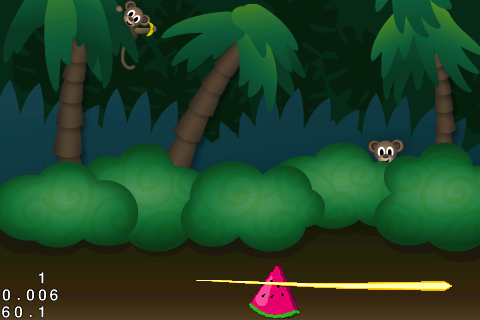## Where To Go From Here?

Here is a sample project with all of the code from the above tutorial.

That's it for Part 2 of the series. In Part 1, you created a Watermelon Textured Polygon that falls to the bottom of the screen. Now, you are able to slice and dice the Watermelon into tiny little pieces with a cool blade.

In the next and last part of the series, you will finally turn everything you have into a complete game!This is a post by iOS Tutorial Team Member Allen Tan, an iOS developer and co-founder at White Widget. You can also find him on and Twitter.